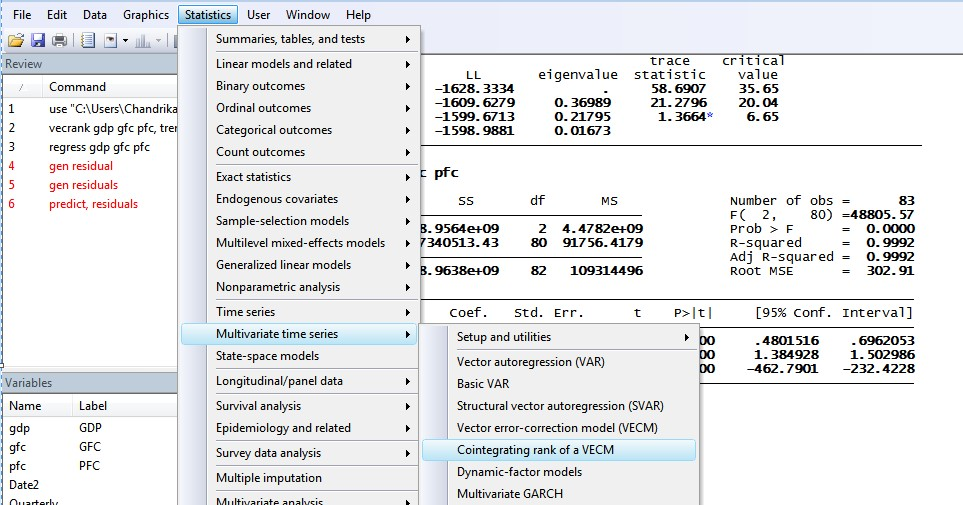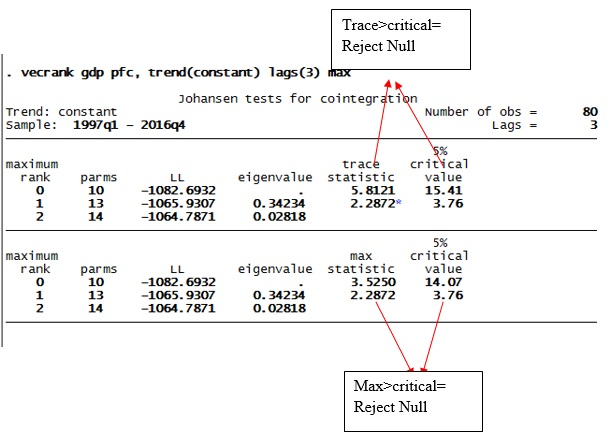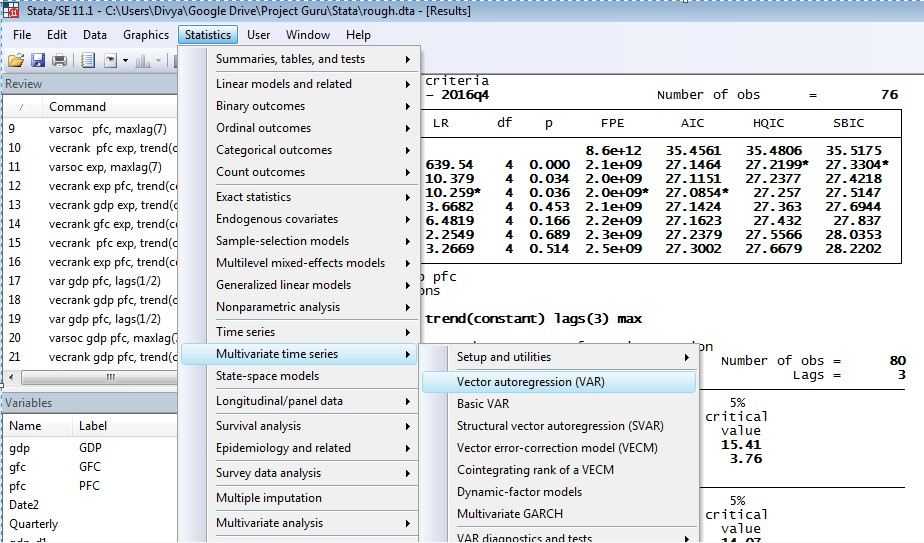# Lag selection and cointegration test in VAR with two variables

The previous article showed that the three-time series values Gross Domestic Product (GDP), Gross Fixed Capital Formation (GFC) and Private Final Consumption (PFC) are non-stationary. Therefore they may have long-term causality. The general assumption, in this case, is that consumption PFC affects GDP, therefore these variables might be cointegrated. Resultantly, they may lead to an estimation of a stationary variable. Johansen cointegration test in Vector Auto Regression (VAR) with two variables will help check the same.

1. Click on ‘Statistics’ on result window
2. Choose ‘Multi-variate Time Series’
3. Click on ‘VAR Diagnostic and Test’
4. Select ‘Lag-order selection statistics’.

The below screen will appear.Figure 1: Steps for lag selection parameters to perform cointegration test in VAR using two variables in STATA

When clicked on ‘lag-order selection statistics’, a varsoc window will open in STATA as shown in figure 2. In the varsoc window, select two components on the main page: the list of dependent variables (GDP and PFC), and the maximum lag order. Here the maximum lag order refers to the maximum lag you want to check for the results.Figure 2: Varsoc window in STATA for lag selection

In ‘Dependent variables’, select the main variables GDP and PFC. After selection for both dropdowns, click on ‘OK’.Figure 3: Varsoc window in STATA for lag selection

After clicking on ‘OK’, the results will appear in the output window (figure 4). Here the STATA command for lag selection parameters is also visible. Use this command alternatively to generate the result.

##### Command
`varsoc gdp pfc, max lag (8)`Figure 4: Results for lag selection parameters for GDP and PFC

The results table will show the number of lags in the first column and a number of parameters. Select the optimal lags, like, Final Prediction Error (FPE), Akaike Information Criterion (AIC), Hannan Quinn Information Parameters (HQIC) and Schwartz Information Parameters (SBIC). STATA will compute four information parameters as well as a sequence of likelihood ratio tests.

## Identify the number of lags

To identify the number of lags, select the values showing.

For instance, in the values for FPE, value at lag 3 carries the sign.

Therefore, the lag as per FPE parameters is 3. Following the same rule, the lag as per AIC is also 3, and as per HQIC and SBIC is 2. To select parameters with optimal lags for VAR, follow the majority. That means if three or four out of four parameters shows the same number of lags (let’s say 3), then take 3 lags. However, in this case, a majority cannot be followed since two parameters show ‘2’ and other two others show ‘3’. Hence, since the number of observations, in this case, is more than 60, follow AIC and FPE parameters. Therefore, the number of lags selected for the present case is 3.

## Johansen cointegration test

Johansen cointegration test, also known as eigenvalue test or trace test, is a likelihood ratio test. There are two tests under Johansen cointegration; maximum eigenvalue test, and trace test. For both test statistics, the initial Johansen test is a test of the null hypothesis of no cointegration against the alternative of cointegration. The null hypothesis for this test differs in case of differing ranks. For clarity, the Johansen cointegration test is performed for variables GDP and PFC. Follow these steps to start (figure below):

1. Click on ‘Statistics’ on ‘Result’ window
2. Select ‘Multivariate Time-series’
3. Select ‘Co-integrating rank of a VECM’.Figure 5: STATA pathway for Johansen cointegration test

‘Vecrank’ window will open in STATA (figure below). In this window, select values for two drop-down options; dependent variables and maximum lags for underlining VAR Model.Figure 6: Vecrank window on STATA for Johansen co-integration analysis

In ‘Dependent variables’ option, select two-time series variables GDP and PFC. Since co-integration analysis takes the case of non-stationary variables to check for causality, take GDP and PFC instead of their first differences. Then select the number of lags. In this case, the lag selected parameters were conducted in a previous analysis, therefore, the number of lags here is 3.

After selecting for lag, click on ‘Reporting’ tab of the vecrank window and click on ‘Report maximum-eigenvalue statistic’ (figure below). Click on ‘OK’.Figure 7: Reporting page of vecrank window on STATA for Johansen cointegration test in VAR with two variables

The results for Johansen cointegration test will appear in the window (figure below). Here the STATA command of Johansen cointegration test will also appear.Figure 8: Result of Johansen cointegration test in VAR with two variables using STATA

##### Command
`vecrank gdp pfc, trend(constant) max`

The result of the Johansen co-integration test can be interpreted in parts. Converge the focus towards three columns; maximum rank, trace statistics or max statistics and critical values.

### Maximum rank zero

Starting from maximum rank zero, the null and alternative hypotheses are as follows:

• Null Hypothesis: There is no cointegration
• Alternative Hypothesis: There is cointegrationFigure 9: Result of Johansen cointegration test in VAR with two variables

As the figure above shows, at maximum rank zero, the trace statistic (5.8121) do not exceed critical values (15.41). Therefore null hypothesis cannot be rejected. Also, this suggests that the time series variables GDP and PFC are not cointegrated. Similarly, for max statistics, the value 3.5250 does not exceed the critical value of 14.07, thus suggesting a similar result that null hypothesis cannot be rejected. Thus, as per maximum rank 0, GDP and PFC are not cointegrated. Following the above results, apply unrestricted VAR to time series GDP and PFC.

## VAR Model

1. Click on ‘Statistics’
2. Select ‘Multivariate Time Series’
3. Select ‘VAR’

The figure below will appear.Figure 10: VAR pathway in STATA

In ‘Dependent variables’ option, select the two-time series variables GDP and PFC. Next, select the number of lags (figure below). The number of lags for this case is same as the previous analysis, i.e. 3.Figure 11: Dialogue box for VAR with two variables in STATA

The figure below shows the results of VAR test. The results are in two parts. While the first one assumes GDP as a dependent variable, the second one assumes PFC as a dependent variable. Since the aim is to verify the effect of PFC on GDP, the first part is more relevant. As per the results:

1. Only lag 1 of PFC is significantly identified having an effect on GDP.
2. R square for GDP model is also 99% verifying the goodness of fit.
3. Log-likelihood value 1064 is also highest, further indicating consistency.
4. The constant identified in GDP model is also significant with 0 p-value.Figure 12: Results of VAR with two variables in STATA

1. GDP and PFC Johnsen cointegration test indicates that there is no cointegration between the two-time series.
2. VAR model indicates that PFC at lag one has significant effects on GDP.

The next article shows the analysis including an additional time series GFC. The aim is to see how results of Johnsen cointegration test changes when adding GFC as a variable in the VAR model along with GDP and PFC.

### Divya Dhuria

Research analyst at Project Guru
DIvya has a keen interest in policy making and wealth management. She is a Master in Economics from Gokhale Institute of Politics and Economics. She has been trained in the econometric techniques to assess different possible economic relationships. She hascontributed to the working paper on National Rural Health Mission at Institute of economic growth, Delhi. Along with academical growth, she likes to explore and visit different places in her spare time.

#### Latest posts by Divya Dhuria (see all)

Discussions

1.JOHN RIVEROS

Greetings, I’d first like to thank to the authors of making this kind of tutorials for Stata and the adecuate interpretations of the outputs. Second… I’ve got a question.

Why are you using VAR models when the variables in levels are non-stationary? Just because Johansen test indicated that there are no cointegration ecuations?

I’ve read a little about VAR and non-stationary variables and seems that it’s not good to use VAR in such variables for the spurious outcome.

Thank you.
John R

2.cba pan

Hi,
I am wondering how I can perform Johansen fisher cointegration test for the panel data including the lag selection. Thank you.
best,
cba

3.Harini

This was very helpful to perform the analysis in my Thesis. Thanks.We are looking for candidates who have completed their master's degree or Ph.D. Click here to know more about our vacancies.